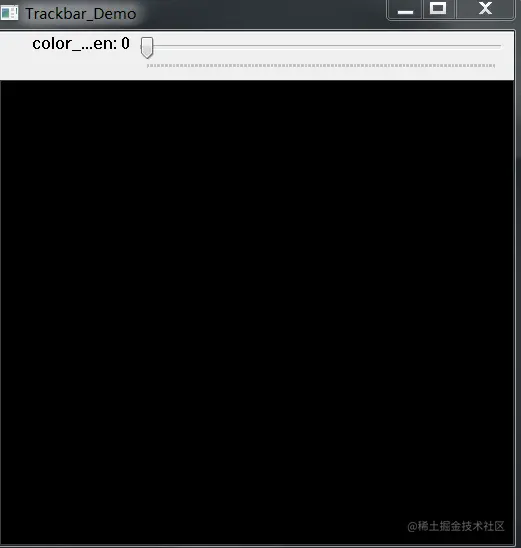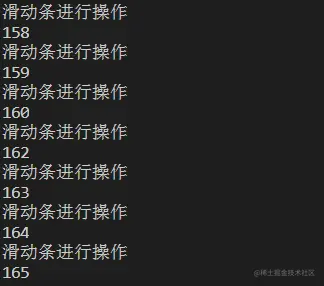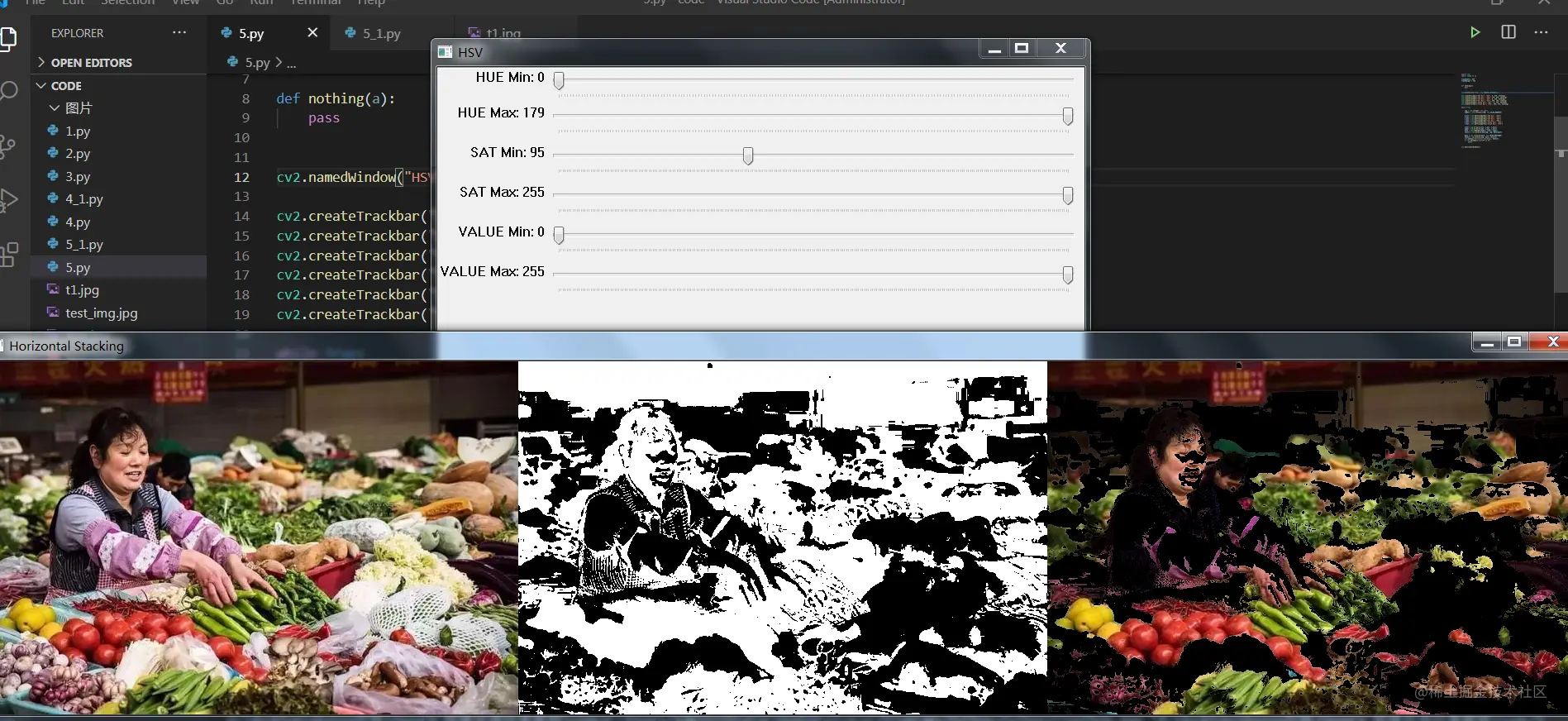# Fanwai 5 Detailed description of slider in Python opencv and solutions to common problems

2022-01-30 19:58:39

「 This is my participation 11 The fourth of the yuegengwen challenge 1 God , Check out the activity details ：2021 One last more challenge

## The way this series is written

This series of column writing will adopt the original question and answer writing form , Quickly let you learn OpenCV My primary 、 intermediate 、 Advanced knowledge .

## 5. Python OpenCV Detailed description of the sliding bar in and solutions to common problems

The slider is in OpenCV The usage scenarios in related cases are very rich , Many times, it is necessary to obtain the relevant parameters with the help of the slider .

Master the slider , Just master cv2.createTrackbar And cv2.getTrackbarPos Two functions .

Prototypes of the above two functions , You can go directly through help Function lookup , This series of columns only give a brief description of the function prototype

``````createTrackbar(trackbarName, windowName, value, count, onChange) -> None
getTrackbarPos(trackbarname, winname) -> retval
Copy code ``````

among cv2.createTrackbar The function parameters are richer ,cv2.getTrackbarPos A function is its child .

Next, through a simple case , Realize the basic application of sliding bar , Drag to change the color of a rectangle in the window .

``````import cv2
import numpy as np

cv2.namedWindow("Trackbar_Demo", cv2.WINDOW_NORMAL)

#  In order to occupy the parameter position in the slider

def nothing():
pass

ret = np.zeros([512, 512, 3], np.uint8)
#  Create a slider
cv2.createTrackbar("color_green", "Trackbar_Demo", 0, 255, nothing)

while 1:
#  Get the value of the slider
color_green = cv2.getTrackbarPos("color_green", "Trackbar_Demo")
cv2.rectangle(ret, (100, 100), (200, 200), (0, color_green, 0), -1)
cv2.imshow("Trackbar_Demo", ret)
if cv2.waitKey(1) & 0xFF == ord('q'):
break

cv2.destroyAllWindows()
Copy code ``````

If the following occurs during code writing BUG, Lack of parameter ：

``````TypeError: function takes exactly 5 arguments (4 given)
Copy code ``````

The BUG Express cv2.createTrackbar The last... Is missing from the function onChange Parameters , Add this parameter , The function body pointed to by the parameter can be empty .

The dynamic picture effect after running the code is as follows ：### cv2.createTrackbar Function onChange Solutions to parameter related problems

Search all Chinese materials on the Internet , You will find a knowledge point in cv2.createTrackbar Function is often ignored in the application of , So this is onChange Parameters , The official description of this parameter is as follows ：

Pointer to the function to be called every time the slider changes position. This function should be prototyped as `void Foo(int,void*);` , where the first parameter is the trackbar position and the second parameter is the user data (see the next parameter). If the callback is the NULL pointer, no callbacks are called, but only `value` is updated.

Here is a detail to pay attention to , stay OpenCV C++ At the time of writing , There is one more parameter in this function , be called userdata , in other words C++ The prototype of this function in is as follows ：

``int createTrackbar(const String& trackbarname, const String& winname, int* value, int count, TrackbarCallback onChange=0, void* userdata=0)  Copy code ``

however Python It's integrated , The operation of two data values is completed through one parameter , So, in fact, every time we drag the slider , Can be operated accordingly .

Modify the code as follows , Space relationship , Modify only nothing Function part , Run the code and the following figure will appear ：

``````#  The callback function of each step of the slider
def nothing(x):
print(" Slide bar to operate ")
print(x)
Copy code ``````I can even adjust the code logic directly , Put the rectangle drawing operation into the callback function of the scroll bar , The specific code and description are as follows ：

``````import cv2
import numpy as np

cv2.namedWindow("Trackbar_Demo", cv2.WINDOW_NORMAL)

#  The callback function of each step of the slider
def nothing(x):
print(" Slide bar to operate ")
cv2.rectangle(ret, (100, 100), (200, 200), (0, x, 0), -1)

ret = np.zeros([512, 512, 3], np.uint8)
#  Create a slider
cv2.createTrackbar("color_green", "Trackbar_Demo", 0, 255, nothing)

# color_green = cv2.getTrackbarPos("color_green", "Trackbar_Demo")
while True:
cv2.imshow("Trackbar_Demo", ret)
if cv2.waitKey(1) & 0xFF == ord('q'):
break

cv2.destroyAllWindows()
Copy code ``````

With this logic , We can design the slider as a switch , Drag to different values , Can present different effects .

For more details , You can continue to read the relevant contents of the manual , Access address

### OpenCV Split the slider and picture window

In the actual coding process , It is recommended to split the slider window and the picture window for display , It is convenient to adjust various parameters .

For example, the following manual parameter adjustment realizes the operation of binarization （ Subsequent articles will cover ）.

``````import cv2
import numpy as np

def nothing(a):
pass

cv2.namedWindow("HSV",cv2.WINDOW_AUTOSIZE)

cv2.createTrackbar("HUE Min", "HSV", 0, 255, nothing)
cv2.createTrackbar("HUE Max", "HSV", 180, 255, nothing)
cv2.createTrackbar("SAT Min", "HSV", 255, 255, nothing)
cv2.createTrackbar("SAT Max", "HSV", 43, 255, nothing)
cv2.createTrackbar("VALUE Min", "HSV", 255, 255, nothing)
cv2.createTrackbar("VALUE Max", "HSV", 46, 255, nothing)

while True:

imgHsv = cv2.cvtColor(img, cv2.COLOR_BGR2HSV)

h_min = cv2.getTrackbarPos("HUE Min", "HSV")
h_max = cv2.getTrackbarPos("HUE Max", "HSV")
s_min = cv2.getTrackbarPos("SAT Min", "HSV")
s_max = cv2.getTrackbarPos("SAT Max", "HSV")
v_min = cv2.getTrackbarPos("VALUE Min", "HSV")
v_max = cv2.getTrackbarPos("VALUE Max", "HSV")

lower = np.array([h_min, s_min, v_min])
upper = np.array([h_max, s_max, v_max])
mask = cv2.inRange(imgHsv, lower, upper)

hStack = np.hstack([img, mask, result])
cv2.imshow('Horizontal Stacking', hStack)
if cv2.waitKey(1) & 0xFF == 27:
break

cv2.destroyAllWindows()
Copy code ``````

After the above code runs , Will present a very convenient operation , Drag a window , The corresponding changes can be dynamically presented in another window .There is a note when coding this place , namely cv2.namedWindow("HSV", cv2.WINDOW_AUTOSIZE) Function part , Please use cv2.WINDOW_AUTOSIZE value , If you do not use this value , It's easy to cause the slider window to be out of order , Or uneven distribution of window parameters .

Finally, another function of the slider is emphasized again , Change button . After all, OpenCV Button function does not exist in , Using a slider with a fixed value can effectively solve this problem .

The use code is as follows ：

``````cv2.createTrackbar(switch,'image',0,1,nothing)
If this value is not used , It's easy to cause the slider window to be out of order , Or uneven distribution of window parameters .

Finally, another function of the slider is emphasized again , Change button . After all,  OpenCV  Button function does not exist in , Using a slider with a fixed value can effectively solve this problem .

The use code is as follows ：

```python
cv2.createTrackbar(switch,'image',0,1,nothing)
Copy code ``````